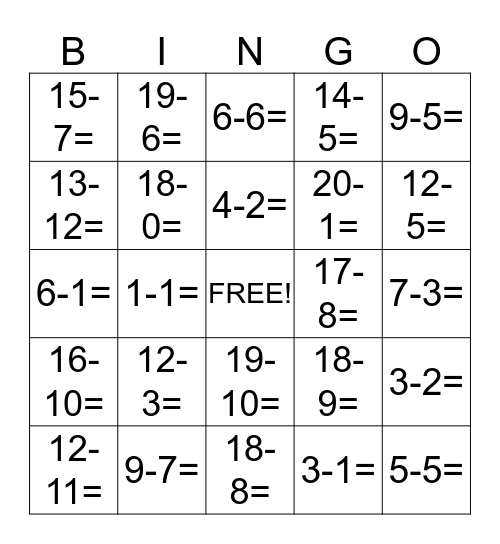# aftrekken tot 20This bingo card has a free space and 48 words: 20-5=, 5-5=, 5-4=, 18-8=, 12-3=, 4-4=, 19-6=, 14-9=, 13-12=, 11-11=, 18-0=, 14-5=, 3-2=, 18-9=, 11-5=, 6-3=, 4-2=, 17-8=, 9-9=, 9-5=, 1-1=, 3-1=, 15-7=, 20-1=, 14-13=, 12-5=, 12-11=, 13-13=, 19-10=, 16-10=, 16-8=, 5-2=, 9-9=, 9-8=, 9-7=, 6-5=, 6-4=, 7-3=, 7-4=, 7-5=, 7-7=, 7-0=, 6-6=, 6-5=, 6-1=, 18-7=, 14-5= and 15-4=.

⚠ This card has duplicate items: 14-5= (2), 9-9= (2), 6-5= (2)

## Play Online